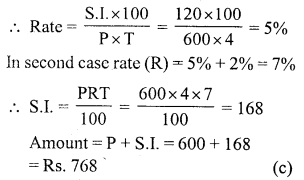# RS Aggarwal Class 7 Solutions Chapter 12 Simple Interest Ex 12B

In this chapter, we provide RS Aggarwal Solutions for Class 7 Chapter 12 Simple Interest Ex 12B for English medium students, Which will very helpful for every student in their exams. Students can download the latest RS Aggarwal Solutions for Class 7 Chapter 12 Simple Interest Ex 12B Maths pdf, free RS Aggarwal Solutions Class 7 Chapter 12 Simple Interest Ex 12B Maths book pdf download. Now you will get step by step solution to each question.

### RS Aggarwal Solutions for Class 7 Chapter 12 Simple Interest Ex 12B Download PDF

Question 1.
Solution:
Principal (P) = Rs. 6250
Rate of (R) = 4% p.a.
Period (T) = 6 months = 12 yearQuestion 2.
Solution:
Amount (A) = Rs. 3605
Rate (R) = 5% p.a.Question 3.
Solution:
Let sum (P) = Rs. 100Question 4.
Solution:
Principal (P) = Rs. 8000
Amount (A) – Rs. 8360
S.I. = A – P = Rs. 8360 – Rs. 8000 = Rs. 360
Rate (R) = 6% p.a.Question 5.
Solution:
Let sum (P) = Rs. 100
Then amount (A) = Rs. 100 x 2 = Rs. 200
S.I. = A – P = Rs. 200 – Rs. 100 = Rs. 100
Period (T) = 10 yearsQuestion 6.
Solution:
S.I. = Rs. x
Rate (R) = x% p.a.
Time (T) = x yearRs. 100x (c)

Question 7.
Solution:
Let sum (P) = Rs. 100Question 8.
Solution:
A’s principal (P) = Rs. 8000
Rate (R) = 12% p.a.
B’s principal = Rs. 9100
Rate = 10%
Let after x years, then amount will be equalQuestion 9.
Solution:
Amount (A) = Rs. 720
Principal (P) = Rs. 600
S.I. = A – P = Rs. 720 – 600 = Rs. 120
Period (T) = 4 yearsQuestion 10.
Solution:Question 11.
Solution:
Let sum (P) = Rs. 100
Their S.I. = 0.125 of Rs. 100Question 12.
Solution:
S.I. = Rs. 210All Chapter RS Aggarwal Solutions For Class 7 Maths

—————————————————————————–

All Subject NCERT Exemplar Problems Solutions For Class 7

All Subject NCERT Solutions For Class 7

*************************************************

I think you got complete solutions for this chapter. If You have any queries regarding this chapter, please comment on the below section our subject teacher will answer you. We tried our best to give complete solutions so you got good marks in your exam.

If these solutions have helped you, you can also share rsaggarwalsolutions.in to your friends.